Next: About this document ... Up: lab_template Previous: lab_template

Subsections

# Double integrals with Maple

## Purpose

The purpose of this lab is to acquaint you with using Maple to do double integrals.

## Getting Started

To assist you, there is a worksheet associated with this lab that contains examples. You can copy that worksheet to your home directory with the following command, which must be run in a terminal window, not in Maple.

cp ~bfarr/Doubleint_start.mws ~


You can copy the worksheet now, but you should read through the lab before you load it into Maple. Once you have read to the exercises, start up Maple, load the worksheet Doubleint_start.mws, and go through it carefully. Then you can start working on the exercises.

## Background

### Volumes from double integrals

Suppose that
is a rectangular region in the the
plane, and that
is a continuous, non-negative function on. Then the volume of the solid aboveand below
is given by the double integral
You learned in class that such integrals can be evaluated by either of the iterated integrals
or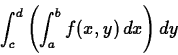where the rectangleis defined by the inequalities
and
.

The worksheet associated with this lab contains examples of how to use Maple to compute double integrals. It also has an example of how to use Maple if the region of integration is of the more complicated form. This is the case where the base of the solid is not rectangular, but is bounded by two curves
and. Ifis as before, then the volume of the solid above
and belowis given by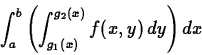The other case, where the regionis
-simple can also be handled using Maple, and there is an example in the worksheet.

## Exercises

1. Use Maple to compute the following double integrals.

1.2.
3.
4.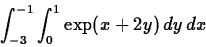2. Letbe the region in theplane containing the origin and bounded by the two curvesand
. Computewhere
. Include a plot of the regionin your worksheet.

3. Consider the following integral
where the regionis bounded by
,
, and
. First, plot the region. Then compute the integral usingas the inner variable of integration. Repeat the calculuation using
as the inner variable of integration. You should get the same answer.

4. Use a double integral to find the volume of the region beneath the plane with equationand above the triangle with vertices
,
, and. Include a plot of the region in the
plane.Next: About this document ... Up: lab_template Previous: lab_template
William W. Farr
2002-09-20